The OpenFOAM Foundation
solidChemistryModel< CompType, SolidThermo > Class Template Referenceabstract

Extends base solid chemistry model by adding a thermo package, and ODE functions. Introduces chemistry equation system and evaluation of chemical source terms. More...

Inheritance diagram for solidChemistryModel< CompType, SolidThermo >: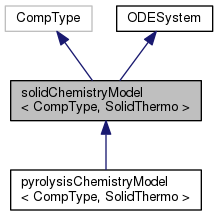[legend]
Collaboration diagram for solidChemistryModel< CompType, SolidThermo >: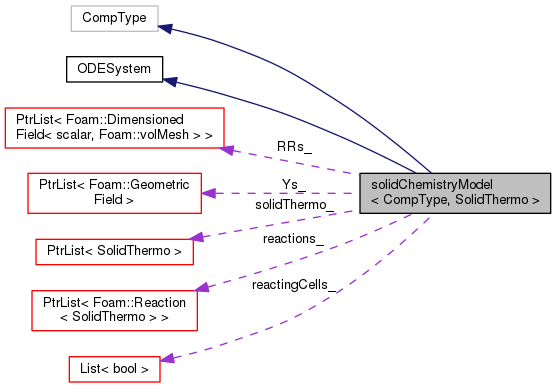[legend]

## Public Member Functions

TypeName ("solidChemistryModel")
Runtime type information. More...

solidChemistryModel (const fvMesh &mesh, const word &phaseName)
Construct from mesh and phase name. More...

virtual ~solidChemistryModel ()
Destructor. More...

const PtrList< Reaction< SolidThermo > > & reactions () const
The reactions. More...

label nReaction () const
The number of reactions. More...

virtual scalarField omega (const scalarField &c, const scalar T, const scalar p, const bool updateC0=false) const =0
dc/dt = omega, rate of change in concentration, for each species More...

virtual scalar omega (const Reaction< SolidThermo > &r, const scalarField &c, const scalar T, const scalar p, scalar &pf, scalar &cf, label &lRef, scalar &pr, scalar &cr, label &rRef) const =0
Return the reaction rate for reaction r and the reference. More...

virtual scalar omegaI (label iReaction, const scalarField &c, const scalar T, const scalar p, scalar &pf, scalar &cf, label &lRef, scalar &pr, scalar &cr, label &rRef) const =0
Return the reaction rate for iReaction and the reference. More...

virtual void calculate ()=0
Calculates the reaction rates. More...

const DimensionedField< scalar, volMesh > & RRs (const label i) const
Return const access to the chemical source terms for solids. More...

tmp< DimensionedField< scalar, volMesh > > RRs () const

virtual scalar solve (const scalar deltaT)=0
Solve the reaction system for the given time step. More...

virtual scalar solve (const scalarField &deltaT)
Solve the reaction system for the given time step. More...

virtual tmp< volScalarFieldtc () const
Return the chemical time scale. More...

virtual tmp< volScalarFieldSh () const
Return source for enthalpy equation [kg/m/s3]. More...

virtual tmp< volScalarFielddQ () const
Return the heat release, i.e. enthalpy/sec [m2/s3]. More...

virtual label nEqns () const =0
Number of ODE's to solve. More...

virtual void derivatives (const scalar t, const scalarField &c, scalarField &dcdt) const =0
Calculate the derivatives in dydx. More...

virtual void jacobian (const scalar t, const scalarField &c, scalarField &dcdt, scalarSquareMatrix &dfdc) const =0
Calculate the Jacobian of the system. More...

virtual void solve (scalarField &c, scalar &T, scalar &p, scalar &deltaT, scalar &subDeltaT) const =0Public Member Functions inherited from ODESystem
ODESystem ()
Construct null. More...

virtual ~ODESystem ()
Destructor. More...

## Protected Member Functions

PtrList< DimensionedField< scalar, volMesh > > & RRs ()
Write access to source terms for solids. More...

void setCellReacting (const label celli, const bool active)
Set reacting status of cell, celli. More...

## Protected Attributes

PtrList< volScalarField > & Ys_
Reference to solid mass fractions. More...

const PtrList< Reaction< SolidThermo > > & reactions_
Reactions. More...

const PtrList< SolidThermo > & solidThermo_
Thermodynamic data of solids. More...

label nSolids_
Number of solid components. More...

label nReaction_
Number of solid reactions. More...

PtrList< DimensionedField< scalar, volMesh > > RRs_
List of reaction rate per solid [kg/m3/s]. More...

List< bool > reactingCells_
List of active reacting cells. More...

## Detailed Description

### template<class CompType, class SolidThermo> class Foam::solidChemistryModel< CompType, SolidThermo >

Extends base solid chemistry model by adding a thermo package, and ODE functions. Introduces chemistry equation system and evaluation of chemical source terms.

Source files

Definition at line 60 of file solidChemistryModel.H.

## Constructor & Destructor Documentation

 solidChemistryModel ( const fvMesh & mesh, const word & phaseName )

Construct from mesh and phase name.

Definition at line 34 of file solidChemistryModel.C.

Here is the call graph for this function: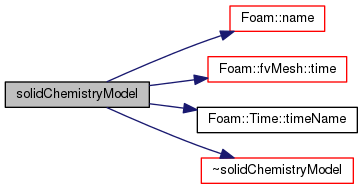~solidChemistryModel ( )
virtual

Destructor.

Definition at line 89 of file solidChemistryModel.C.

Here is the call graph for this function: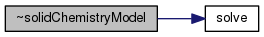Here is the caller graph for this function: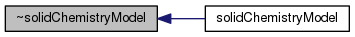## Member Function Documentation

 Foam::PtrList< Foam::DimensionedField< Foam::scalar, Foam::volMesh > > & RRs ( )
inlineprotected

Write access to source terms for solids.

Definition at line 32 of file solidChemistryModelI.H.

Here is the caller graph for this function: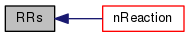void setCellReacting ( const label celli, const bool active )
protected

Set reacting status of cell, celli.

Definition at line 189 of file solidChemistryModel.C.

Referenced by solidChemistryModel< CompType, SolidThermo >::dQ().

Here is the caller graph for this function: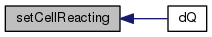TypeName ( "solidChemistryModel< CompType, SolidThermo >" )

Runtime type information.

 const Foam::PtrList< Foam::Reaction< SolidThermo > > & reactions ( ) const
inline

The reactions.

Definition at line 39 of file solidChemistryModelI.H.

Here is the call graph for this function: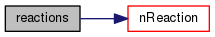Foam::label nReaction ( ) const
inline

The number of reactions.

Definition at line 48 of file solidChemistryModelI.H.

Here is the call graph for this function: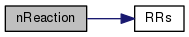Here is the caller graph for this function: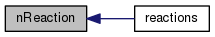virtual scalarField omega ( const scalarField & c, const scalar T, const scalar p, const bool updateC0 = `false` ) const
pure virtual

dc/dt = omega, rate of change in concentration, for each species

Implemented in pyrolysisChemistryModel< CompType, SolidThermo, GasThermo >.

 virtual scalar omega ( const Reaction< SolidThermo > & r, const scalarField & c, const scalar T, const scalar p, scalar & pf, scalar & cf, label & lRef, scalar & pr, scalar & cr, label & rRef ) const
pure virtual

Return the reaction rate for reaction r and the reference.

species and charateristic times

Implemented in pyrolysisChemistryModel< CompType, SolidThermo, GasThermo >.

 virtual scalar omegaI ( label iReaction, const scalarField & c, const scalar T, const scalar p, scalar & pf, scalar & cf, label & lRef, scalar & pr, scalar & cr, label & rRef ) const
pure virtual

Return the reaction rate for iReaction and the reference.

species and charateristic times

Implemented in pyrolysisChemistryModel< CompType, SolidThermo, GasThermo >.

 virtual void calculate ( )
pure virtual

Calculates the reaction rates.

Implemented in pyrolysisChemistryModel< CompType, SolidThermo, GasThermo >.

 const Foam::DimensionedField< Foam::scalar, Foam::volMesh > & RRs ( const label i ) const
inline

Return const access to the chemical source terms for solids.

Definition at line 57 of file solidChemistryModelI.H.

 Foam::tmp< Foam::DimensionedField< Foam::scalar, Foam::volMesh > > RRs ( ) const
inline

Definition at line 67 of file solidChemistryModelI.H.

References Foam::dimMass, Foam::dimTime, Foam::dimVolume, mesh, tmp< T >::ref(), and timeName.

Here is the call graph for this function: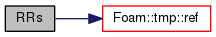virtual scalar solve ( const scalar deltaT )
pure virtual

Solve the reaction system for the given time step.

and return the characteristic time

Implemented in pyrolysisChemistryModel< CompType, SolidThermo, GasThermo >.

Here is the caller graph for this function: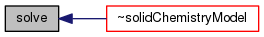Foam::scalar solve ( const scalarField & deltaT )
virtual

Solve the reaction system for the given time step.

and return the characteristic time

Definition at line 97 of file solidChemistryModel.C.

References NotImplemented.

 Foam::tmp< Foam::volScalarField > tc ( ) const
virtual

Return the chemical time scale.

Definition at line 108 of file solidChemistryModel.C.

References NotImplemented.

 Foam::tmp< Foam::volScalarField > Sh ( ) const
virtual

Return source for enthalpy equation [kg/m/s3].

Definition at line 117 of file solidChemistryModel.C.

References Foam::dimEnergy, Foam::dimTime, Foam::dimVolume, forAll, tmp< T >::ref(), and Sh.

Here is the call graph for this function: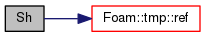Foam::tmp< Foam::volScalarField > dQ ( ) const
virtual

Return the heat release, i.e. enthalpy/sec [m2/s3].

Definition at line 157 of file solidChemistryModel.C.

Here is the call graph for this function: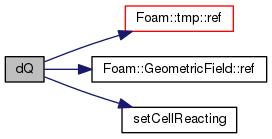virtual label nEqns ( ) const
pure virtual

Number of ODE's to solve.

Implements ODESystem.

Implemented in pyrolysisChemistryModel< CompType, SolidThermo, GasThermo >.

 virtual void derivatives ( const scalar x, const scalarField & y, scalarField & dydx ) const
pure virtual

Calculate the derivatives in dydx.

Implements ODESystem.

Implemented in pyrolysisChemistryModel< CompType, SolidThermo, GasThermo >.

 virtual void jacobian ( const scalar x, const scalarField & y, scalarField & dfdx, scalarSquareMatrix & dfdy ) const
pure virtual

Calculate the Jacobian of the system.

Need by the stiff-system solvers

Implements ODESystem.

Implemented in pyrolysisChemistryModel< CompType, SolidThermo, GasThermo >.

 virtual void solve ( scalarField & c, scalar & T, scalar & p, scalar & deltaT, scalar & subDeltaT ) const
pure virtual

## Member Data Documentation

 PtrList& Ys_
protected

Reference to solid mass fractions.

Definition at line 77 of file solidChemistryModel.H.

 const PtrList >& reactions_
protected

Reactions.

Definition at line 80 of file solidChemistryModel.H.

 const PtrList& solidThermo_
protected

Thermodynamic data of solids.

Definition at line 83 of file solidChemistryModel.H.

 label nSolids_
protected

Number of solid components.

Definition at line 86 of file solidChemistryModel.H.

 label nReaction_
protected

Number of solid reactions.

Definition at line 89 of file solidChemistryModel.H.

 PtrList > RRs_
protected

List of reaction rate per solid [kg/m3/s].

Definition at line 92 of file solidChemistryModel.H.

 List reactingCells_
protected

List of active reacting cells.

Definition at line 95 of file solidChemistryModel.H.

The documentation for this class was generated from the following files: